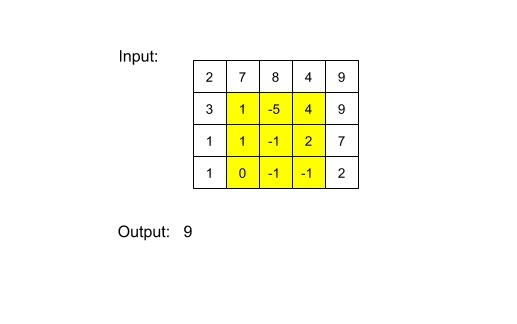# Sub-Matrix with Sum Zero

Posted: 4 Jan, 2021
Difficulty: Moderate

## PROBLEM STATEMENT

#### For example :#### If there is no sub-matrix with sum equal to 0, then return 0.

##### Input Format
``````The first line of input contains an integer 'T' representing the number of test cases. Then the test cases follow.

The first line of each test case contains two single-spaced integers 'N' and 'M', representing the number of rows and columns of the matrix, respectively.

The next 'N' lines contain 'M' single-spaced integers denoting matrix elements.
``````
##### Output Format:
``````For each test case, in the first line, print an integer denoting the size of the largest sub-matrix with sum equal to 0.

The output of each test case is printed in a separate line.
``````
##### Note:
``````You do not need to print anything, it has already been taken care of. Just implement the given function.
``````
##### Constraints:
``````1 <= T <= 10
1 <= N, M <= 50
-10^5 <= data <= 10^5,

Where ‘data’ is the value of the elements of the matrix.

Time Limit: 1 sec
``````Approach 1

In this approach, we will find all possible sub-matrices and check whether it has sum equal to zero or not.

We will define a sub-matrix using 4 variables ('R1', ‘C1’, ‘R2’, ‘C2’). ('R1', ‘C1’) denotes the top-left corner of the sub-matrix and ('R2', ‘C2’) denotes the bottom-right corner of the sub-matrix.

For ('R1': 0 to ‘N-1’){

For ('C1': 0 to 'M-1'){

For('R2': ‘R1’ to ‘N-1’){

For ('C2': 'C2' to 'M-1'){

Find the sum of sub-matrix ('R1', ‘C1’, ‘R2’, ‘C2’)

}

}

}

}

For finding the sum of a sub-matrix, we will iterate on the elements of the sub-matrix using two loops.